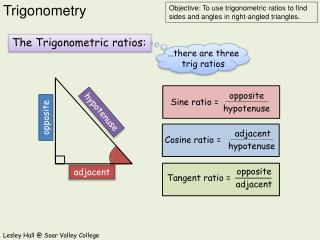Download PresentationThe Trigonometric ratios:# The Trigonometric ratios: - PowerPoint PPT Presentation

Download Presentation##### The Trigonometric ratios:

Download Policy: Content on the Website is provided to you AS IS for your information and personal use and may not be sold / licensed / shared on other websites without getting consent from its author. While downloading, if for some reason you are not able to download a presentation, the publisher may have deleted the file from their server.

- - - - - - - - - - - - - - - - - - - - - - - - - - - E N D - - - - - - - - - - - - - - - - - - - - - - - - - - -
##### Presentation Transcript

1. The Trigonometric ratios: …there are three trig ratios opposite Sine ratio = hypotenuse opposite hypotenuse adjacent Cosine ratio = hypotenuse adjacent opposite Tangent ratio = adjacent

2. Find angle ABC. Choose a ratio. Label the sides. A opposite sin x0 = hypotenuse opp adj Sin = 8 hyp Cos = opp hyp sin x0 = hyp 12 12cm 8cm x0 sin-1 sin 0.6666 = x x = 420 B C opp Tan = adj

3. Find angle ABC. Choose a ratio. Label the sides. adjacent 10cm B A adj cos x0 = hypotenuse x opp adj Sin = 10 Cos = hyp hyp cos x0 = 18 18cm x0 cos cos-1 0.5555 = hyp x = 560 opp Tan = adj C

4. Find the length of side BC. Label the sides. Choose a ratio. B adjacent cos 640 = hypotenuse opp 640 hyp adj Sin = Cos = hyp 15cm hyp x x adj cos 640 = 15 x A C x cos 640 x = 15 opp Tan = adj 6.6cm = x

5. Find the length of side AB. Choose a ratio. Label the sides. opp x opposite A B tan 580 = adjacent opp adj Sin = Cos = hyp hyp x adj tan 580 = 11 11cm x x tan 580 x = 11 opp 580 Tan = adj 17.6cm = x C

6. Find the length of side AB. Choose a ratio. Label the sides. opposite A sin 270 = hypotenuse opp opp adj x Sin = Cos = hyp hyp 7cm hyp 7 sin 270 = x 270 C B 7 = sin 270 = x opp Tan = adj x = 15.4cm

7. Find the length of side BC. Choose a ratio. Label the sides. B opposite tan 390 = adjacent 390 opp adj Sin = Cos = hyp hyp 5.8 tan 390 = x adj x 5.8 = tan 390 = x opp Tan = adj x = 7.2cm A C 5.8cm opp